## Tuesday, April 28, 2015

### Informed priors for Bayesian comparison of two groups

The BEST programs, for Bayesian estimation of two groups, were written with generic vague priors only minimally informed by the scale of the data. Here are new versions of the programs that are better suited for specifying informed priors.

A little background: Suppose we have two groups of metric data. In each group the data seem to be unimodal and symmetrically distributed, but perhaps with outliers relative to a normal distribution. So we chose to describe the groups with possibly heavy-tailed t distributions, but using the same normality (df) parameter for both groups because outliers are rare (and it's the outliers that most influence the normality parameter). Thus there are five parameters to be estimated: μ1, σ1, μ2, σ2, and the normality parameter ν.

The original BEST program put simplistic broad priors on the five parameters. For example, σ1 was given a broad uniform prior extending across a range that far exceeded any plausible estimate for the scale of the data. It was explained how to make basic modifications of the prior in Appendix B of the article describing the programs. But the programs really were not intended for easy expression of informed priors. In the post I present a version of the BEST programs that are much easier for expression of informed priors.

In the new version, each parameter is given its own line of code for expressing its prior, so that each can be individually set.

• Each μ is given a normal prior with its own (constant) mean and standard deviation.
• Each σ is given a gamma prior with its own (constant) shape and rate. The shape and rate are derived from the desired mode and standard deviation of the gamma distribution.
• The normality parameter ν is given a gamma prior with its own (constant) shape and rate. The shape and rate are derived from the desired mean and standard deviation of the gamma distribution.

Pages 236-239 of DBDA2E explain the gamma distribution and formulas for converting the desired mode or mean and standard deviation to corresponding shape and rate values. In particular, it defines the R functions gammaShRaFromModeSD and gammaShRaFromMeanSD that convert desired mean or mode and SD to corresponding shape and rate parameters.

The JAGS model specification is shown below. To understand it, note that the metric data values are y[i] and the corresponding group membership is specified by x[i].

model {
for ( i in 1:Ntotal ) {
y[i] ~ dt( mu[x[i]] , 1/sigma[x[i]]^2 , nu )
}
mu ~ dnorm( muM1 , 1/muSD1^2 )  # prior for mu
sigma ~ dgamma( Sh1 , Ra1 )     # prior for sigma
mu ~ dnorm( muM2 , 1/muSD2^2 )  # prior for mu
sigma ~ dgamma( Sh2 , Ra2 )     # prior for sigma
nu <- nuMinusOne+1
nuMinusOne ~ dgamma( ShNu , RaNu ) # prior for nu
}

To set the prior constants so that they are broad on the scale of the data, I use the following (somewhat arbitrary) settings:

muM1 = mean(y)  # centered on the data
muSD1 = sd(y)*5 # broad relative to the scale of the data

Sh1 = gammaShRaFromModeSD( mode=sd(y) , sd=sd(y)*5 )$shape Ra1 = gammaShRaFromModeSD( mode=sd(y) , sd=sd(y)*5 )$rate

muM2 = mean(y)
muSD2 = sd(y)*5

Sh2 = gammaShRaFromModeSD( mode=sd(y) , sd=sd(y)*5 )$shape Ra2 = gammaShRaFromModeSD( mode=sd(y) , sd=sd(y)*5 )$rate

ShNu = gammaShRaFromMeanSD( mean=29 , sd=29 )$shape RaNu = gammaShRaFromMeanSD( mean=29 , sd=29 )$rate

Notice that the gamma prior for nuMinusOne is exactly the exponential prior used in the original program when its mean=29 and sd=29.

When the new program is run with the broad priors specified above, it gives output virtually identical to the original program for any modest amount of data.

Suppose now that you want to specify a strong prior on the first group, such that you want μ1 to be very nearly 100 and σ1 to be very nearly 15. You could specify that as

muM1 = 15
muSD1 = 1 # very small uncertainty

Sh1 = gammaShRaFromModeSD( mode=15 , sd=1 )$shape Ra1 = gammaShRaFromModeSD( mode=15 , sd=1 )$rate

But it doesn't often make sense to just arbitrary put strong constraints in the prior. Instead, and in general, we would like the prior to resemble a posterior from previous data, starting with a vague proto-prior. Instead of relying on intuition to express an informed prior directly on the parameters, we start with a vague proto-prior and enter some reasonable data that instantiate our prior knowledge. Then we run the Bayesian analysis on the data and observe the resulting posterior distribution. It is the posterior distribution that is now the informed prior for subsequent data analysis. From the posterior distribution of the prior data, just "read off" the mode and sd of the marginal posterior on σ and other parameters. In the program, the MCMC chain is in the matrix mcmcChain, so for example, we can get at the mean and sd of the nu parameter using mean( mcmcChain[,"nu"] ) and sd( mcmcChain[,"nu"] ).

The full scripts are listed below:

BESTgamma.R:

# MODIFIED 4/28/15 WITH GAMMA PRIOR ON SIGMA
# John K. Kruschke
# johnkruschke@gmail.com
# http://www.indiana.edu/~kruschke/BEST/
#
# This program is believed to be free of errors, but it comes with no guarantee!
# The user bears all responsibility for interpreting the results.
#
### ***************************************************************
### ******** SEE FILE BESTgammaExample.R FOR INSTRUCTIONS **************
### ***************************************************************

source("openGraphSaveGraph.R") # graphics functions for Windows, Mac OS, Linux

# Function for shape and rate parameters of gamma. From DBDA2E-utilities.R; see
# p. 238 of DBDA2E. DBDA2E = Doing Bayesian Data Analysis Second Edition,
gammaShRaFromModeSD = function( mode , sd ) {
if ( mode <=0 ) stop("mode must be > 0")
if ( sd <=0 ) stop("sd must be > 0")
rate = ( mode + sqrt( mode^2 + 4 * sd^2 ) ) / ( 2 * sd^2 )
shape = 1 + mode * rate
return( list( shape=shape , rate=rate ) )
}

gammaShRaFromMeanSD = function( mean , sd ) {
if ( mean <=0 ) stop("mean must be > 0")
if ( sd <=0 ) stop("sd must be > 0")
shape = mean^2/sd^2
rate = mean/sd^2
return( list( shape=shape , rate=rate ) )
}

BESTmcmc = function( y1, y2, numSavedSteps=100000, thinSteps=1, showMCMC=FALSE) {
# This function generates an MCMC sample from the posterior distribution.
# Description of arguments:
# showMCMC is a flag for displaying diagnostic graphs of the chains.
#    If F (the default), no chain graphs are displayed. If T, they are.
require(rjags)

#----------------------------------------------------------------------------
# THE MODEL.
modelString = "
model {
for ( i in 1:Ntotal ) {
y[i] ~ dt( mu[x[i]] , 1/sigma[x[i]]^2 , nu )
}
mu ~ dnorm( muM1 , 1/muSD1^2 )  # prior for mu
sigma ~ dgamma( Sh1 , Ra1 )     # prior for sigma
mu ~ dnorm( muM2 , 1/muSD2^2 )  # prior for mu
sigma ~ dgamma( Sh2 , Ra2 )     # prior for sigma
nu <- nuMinusOne+1
nuMinusOne ~ dgamma( ShNu , RaNu ) # prior for nu
}
" # close quote for modelString
# Write out modelString to a text file
writeLines( modelString , con="BESTmodel.txt" )

#------------------------------------------------------------------------------
# THE DATA.
y = c( y1 , y2 ) # combine data into one vector
x = c( rep(1,length(y1)) , rep(2,length(y2)) ) # create group membership code
Ntotal = length(y)
# Specify the data and prior constants in a list, for later shipment to JAGS:
dataList = list(
y = y ,
x = x ,
Ntotal = Ntotal ,
# Below are the specifications for the prior constants. These are generic
# broad priors, but you can replace with informed values if appropriate.
muM1 = mean(y) ,
muSD1 = sd(y)*5 ,
muM2 = mean(y) ,
muSD2 = sd(y)*5 ,
Sh1 = gammaShRaFromModeSD( mode=sd(y) , sd=sd(y)*5 )$shape , Ra1 = gammaShRaFromModeSD( mode=sd(y) , sd=sd(y)*5 )$rate ,
Sh2 = gammaShRaFromModeSD( mode=sd(y) , sd=sd(y)*5 )$shape , Ra2 = gammaShRaFromModeSD( mode=sd(y) , sd=sd(y)*5 )$rate ,
ShNu = gammaShRaFromMeanSD( mean=29 , sd=29 )$shape , RaNu = gammaShRaFromMeanSD( mean=29 , sd=29 )$rate
)

#------------------------------------------------------------------------------
# INTIALIZE THE CHAINS.
# Initial values of MCMC chains based on data:
mu = c( mean(y1) , mean(y2) )
sigma = c( sd(y1) , sd(y2) )
# Regarding initial values in next line: (1) sigma will tend to be too big if
# the data have outliers, and (2) nu starts at 5 as a moderate value. These
# initial values keep the burn-in period moderate.
initsList = list( mu = mu , sigma = sigma , nuMinusOne = 4 )

#------------------------------------------------------------------------------
# RUN THE CHAINS

parameters = c( "mu" , "sigma" , "nu" )     # The parameters to be monitored
adaptSteps = 500               # Number of steps to "tune" the samplers
burnInSteps = 1000
nChains = 3
nIter = ceiling( ( numSavedSteps * thinSteps ) / nChains )
# Create, initialize, and adapt the model:
jagsModel = jags.model( "BESTmodel.txt" , data=dataList , inits=initsList ,
# Burn-in:
cat( "Burning in the MCMC chain...\n" )
update( jagsModel , n.iter=burnInSteps )
# The saved MCMC chain:
cat( "Sampling final MCMC chain...\n" )
codaSamples = coda.samples( jagsModel , variable.names=parameters ,
n.iter=nIter , thin=thinSteps )
# resulting codaSamples object has these indices:
#   codaSamples[[ chainIdx ]][ stepIdx , paramIdx ]

#------------------------------------------------------------------------------
# EXAMINE THE RESULTS
if ( showMCMC ) {
openGraph(width=7,height=7)
show( gelman.diag( codaSamples ) )
effectiveChainLength = effectiveSize( codaSamples )
show( effectiveChainLength )
}

# Convert coda-object codaSamples to matrix object for easier handling.
# But note that this concatenates the different chains into one long chain.
# Result is mcmcChain[ stepIdx , paramIdx ]
mcmcChain = as.matrix( codaSamples )
return( mcmcChain )

} # end function BESTmcmc

#==============================================================================

BESTsummary = function( y1 , y2 , mcmcChain ) {
source("HDIofMCMC.R")
mcmcSummary = function( paramSampleVec , compVal=NULL ) {
meanParam = mean( paramSampleVec )
medianParam = median( paramSampleVec )
dres = density( paramSampleVec )
modeParam = dres$x[which.max(dres$y)]
hdiLim = HDIofMCMC( paramSampleVec )
if ( !is.null(compVal) ) {
pcgtCompVal = ( 100 * sum( paramSampleVec > compVal )
/ length( paramSampleVec ) )
} else {
pcgtCompVal=NA
}
return( c( meanParam , medianParam , modeParam , hdiLim , pcgtCompVal ) )
}
# Define matrix for storing summary info:
summaryInfo = matrix( 0 , nrow=9 , ncol=6 , dimnames=list(
PARAMETER=c( "mu1" , "mu2" , "muDiff" , "sigma1" , "sigma2" , "sigmaDiff" ,
"nu" , "nuLog10" , "effSz" ),
SUMMARY.INFO=c( "mean" , "median" , "mode" , "HDIlow" , "HDIhigh" ,
"pcgtZero" )
) )
summaryInfo[ "mu1" , ] = mcmcSummary( mcmcChain[,"mu"] )
summaryInfo[ "mu2" , ] = mcmcSummary( mcmcChain[,"mu"] )
summaryInfo[ "muDiff" , ] = mcmcSummary( mcmcChain[,"mu"]
- mcmcChain[,"mu"] ,
compVal=0 )
summaryInfo[ "sigma1" , ] = mcmcSummary( mcmcChain[,"sigma"] )
summaryInfo[ "sigma2" , ] = mcmcSummary( mcmcChain[,"sigma"] )
summaryInfo[ "sigmaDiff" , ] = mcmcSummary( mcmcChain[,"sigma"]
- mcmcChain[,"sigma"] ,
compVal=0 )
summaryInfo[ "nu" , ] = mcmcSummary( mcmcChain[,"nu"] )
summaryInfo[ "nuLog10" , ] = mcmcSummary( log10(mcmcChain[,"nu"]) )

N1 = length(y1)
N2 = length(y2)
effSzChain = ( ( mcmcChain[,"mu"] - mcmcChain[,"mu"] )
/ sqrt( ( mcmcChain[,"sigma"]^2 + mcmcChain[,"sigma"]^2 ) / 2 ) )
summaryInfo[ "effSz" , ] = mcmcSummary( effSzChain , compVal=0 )
# Or, use sample-size weighted version:
# effSz = ( mu1 - mu2 ) / sqrt( ( sigma1^2 *(N1-1) + sigma2^2 *(N2-1) )
#                               / (N1+N2-2) )
# Be sure also to change plot label in BESTplot function, below.
return( summaryInfo )
}

#==============================================================================

BESTplot = function( y1 , y2 , mcmcChain , ROPEm=NULL , ROPEsd=NULL ,
ROPEeff=NULL , showCurve=FALSE , pairsPlot=FALSE ) {
# This function plots the posterior distribution (and data).
# Description of arguments:
# y1 and y2 are the data vectors.
# mcmcChain is a list of the type returned by function BTT.
# ROPEm is a two element vector, such as c(-1,1), specifying the limit
#   of the ROPE on the difference of means.
# ROPEsd is a two element vector, such as c(-1,1), specifying the limit
#   of the ROPE on the difference of standard deviations.
# ROPEeff is a two element vector, such as c(-1,1), specifying the limit
#   of the ROPE on the effect size.
# showCurve is TRUE or FALSE and indicates whether the posterior should
#   be displayed as a histogram (by default) or by an approximate curve.
# pairsPlot is TRUE or FALSE and indicates whether scatterplots of pairs
#   of parameters should be displayed.
mu1 = mcmcChain[,"mu"]
mu2 = mcmcChain[,"mu"]
sigma1 = mcmcChain[,"sigma"]
sigma2 = mcmcChain[,"sigma"]
nu = mcmcChain[,"nu"]
if ( pairsPlot ) {
# Plot the parameters pairwise, to see correlations:
openGraph(width=7,height=7)
nPtToPlot = 1000
plotIdx = floor(seq(1,length(mu1),by=length(mu1)/nPtToPlot))
panel.cor = function(x, y, digits=2, prefix="", cex.cor, ...) {
usr = par("usr"); on.exit(par(usr))
par(usr = c(0, 1, 0, 1))
r = (cor(x, y))
txt = format(c(r, 0.123456789), digits=digits)
txt = paste(prefix, txt, sep="")
if(missing(cex.cor)) cex.cor <- 0.8/strwidth(txt)
text(0.5, 0.5, txt, cex=1.25 ) # was cex=cex.cor*r
}
pairs( cbind( mu1 , mu2 , sigma1 , sigma2 , log10(nu) )[plotIdx,] ,
labels=c( expression(mu) , expression(mu) ,
expression(sigma) , expression(sigma) ,
expression(log10(nu)) ) ,
lower.panel=panel.cor , col="skyblue" )
}
source("plotPost.R")
# Set up window and layout:
openGraph(width=6.0,height=8.0)
layout( matrix( c(4,5,7,8,3,1,2,6,9,10) , nrow=5, byrow=FALSE ) )
par( mar=c(3.5,3.5,2.5,0.5) , mgp=c(2.25,0.7,0) )

# Select thinned steps in chain for plotting of posterior predictive curves:
chainLength = NROW( mcmcChain )
nCurvesToPlot = 30
stepIdxVec = seq( 1 , chainLength , floor(chainLength/nCurvesToPlot) )
xRange = range( c(y1,y2) )
xLim = c( xRange-0.1*(xRange-xRange) ,
xRange+0.1*(xRange-xRange) )
xVec = seq( xLim , xLim , length=200 )
maxY = max( dt( 0 , df=max(nu[stepIdxVec]) ) /
min(c(sigma1[stepIdxVec],sigma2[stepIdxVec])) )
# Plot data y1 and smattering of posterior predictive curves:
stepIdx = 1
plot( xVec , dt( (xVec-mu1[stepIdxVec[stepIdx]])/sigma1[stepIdxVec[stepIdx]] ,
df=nu[stepIdxVec[stepIdx]] )/sigma1[stepIdxVec[stepIdx]] ,
ylim=c(0,maxY) , cex.lab=1.75 ,
type="l" , col="skyblue" , lwd=1 , xlab="y" , ylab="p(y)" ,
main="Data Group 1 w. Post. Pred." )
for ( stepIdx in 2:length(stepIdxVec) ) {
lines(xVec, dt( (xVec-mu1[stepIdxVec[stepIdx]])/sigma1[stepIdxVec[stepIdx]] ,
df=nu[stepIdxVec[stepIdx]] )/sigma1[stepIdxVec[stepIdx]] ,
type="l" , col="skyblue" , lwd=1 )
}
histBinWd = median(sigma1)/2
histCenter = mean(mu1)
histBreaks = sort( c( seq( histCenter-histBinWd/2 , min(xVec)-histBinWd/2 ,
-histBinWd ),
seq( histCenter+histBinWd/2 , max(xVec)+histBinWd/2 ,
histBinWd ) , xLim ) )
histInfo = hist( y1 , plot=FALSE , breaks=histBreaks )
yPlotVec = histInfo$density yPlotVec[ yPlotVec==0.0 ] = NA xPlotVec = histInfo$mids
xPlotVec[ yPlotVec==0.0 ] = NA
points( xPlotVec , yPlotVec , type="h" , lwd=3 , col="red" )
text( max(xVec) , maxY , bquote(N==.(length(y1))) , adj=c(1.1,1.1) )
# Plot data y2 and smattering of posterior predictive curves:
stepIdx = 1
plot( xVec , dt( (xVec-mu2[stepIdxVec[stepIdx]])/sigma2[stepIdxVec[stepIdx]] ,
df=nu[stepIdxVec[stepIdx]] )/sigma2[stepIdxVec[stepIdx]] ,
ylim=c(0,maxY) , cex.lab=1.75 ,
type="l" , col="skyblue" , lwd=1 , xlab="y" , ylab="p(y)" ,
main="Data Group 2 w. Post. Pred." )
for ( stepIdx in 2:length(stepIdxVec) ) {
lines(xVec, dt( (xVec-mu2[stepIdxVec[stepIdx]])/sigma2[stepIdxVec[stepIdx]] ,
df=nu[stepIdxVec[stepIdx]] )/sigma2[stepIdxVec[stepIdx]] ,
type="l" , col="skyblue" , lwd=1 )
}
histBinWd = median(sigma2)/2
histCenter = mean(mu2)
histBreaks = sort( c( seq( histCenter-histBinWd/2 , min(xVec)-histBinWd/2 ,
-histBinWd ),
seq( histCenter+histBinWd/2 , max(xVec)+histBinWd/2 ,
histBinWd ) , xLim ) )
histInfo = hist( y2 , plot=FALSE , breaks=histBreaks )
yPlotVec = histInfo$density yPlotVec[ yPlotVec==0.0 ] = NA xPlotVec = histInfo$mids
xPlotVec[ yPlotVec==0.0 ] = NA
points( xPlotVec , yPlotVec , type="h" , lwd=3 , col="red" )
text( max(xVec) , maxY , bquote(N==.(length(y2))) , adj=c(1.1,1.1) )

# Plot posterior distribution of parameter nu:
histInfo = plotPost( log10(nu) , col="skyblue" , # breaks=30 ,
showCurve=showCurve ,
xlab=bquote("log10("*nu*")") , cex.lab = 1.75 , showMode=TRUE ,
main="Normality" ) #  (<0.7 suggests kurtosis)

# Plot posterior distribution of parameters mu1, mu2, and their difference:
xlim = range( c( mu1 , mu2 ) )
histInfo = plotPost( mu1 ,  xlim=xlim , cex.lab = 1.75 ,
showCurve=showCurve ,
xlab=bquote(mu) , main=paste("Group",1,"Mean") ,
col="skyblue" )
histInfo = plotPost( mu2 ,  xlim=xlim , cex.lab = 1.75 ,
showCurve=showCurve ,
xlab=bquote(mu) , main=paste("Group",2,"Mean") ,
col="skyblue" )
histInfo = plotPost( mu1-mu2 , compVal=0 ,  showCurve=showCurve ,
xlab=bquote(mu - mu) , cex.lab = 1.75 , ROPE=ROPEm ,
main="Difference of Means" , col="skyblue" )

# Plot posterior distribution of param's sigma1, sigma2, and their difference:
xlim=range( c( sigma1 , sigma2 ) )
histInfo = plotPost( sigma1 ,  xlim=xlim , cex.lab = 1.75 ,
showCurve=showCurve ,
xlab=bquote(sigma) , main=paste("Group",1,"Std. Dev.") ,
col="skyblue" , showMode=TRUE )
histInfo = plotPost( sigma2 ,  xlim=xlim , cex.lab = 1.75 ,
showCurve=showCurve ,
xlab=bquote(sigma) , main=paste("Group",2,"Std. Dev.") ,
col="skyblue" , showMode=TRUE )
histInfo = plotPost( sigma1-sigma2 ,
compVal=0 ,  showCurve=showCurve ,
xlab=bquote(sigma - sigma) , cex.lab = 1.75 ,
ROPE=ROPEsd ,
main="Difference of Std. Dev.s" , col="skyblue" , showMode=TRUE )

# Plot of estimated effect size. Effect size is d-sub-a from
# Macmillan & Creelman, 1991; Simpson & Fitter, 1973; Swets, 1986a, 1986b.
effectSize = ( mu1 - mu2 ) / sqrt( ( sigma1^2 + sigma2^2 ) / 2 )
histInfo = plotPost( effectSize , compVal=0 ,  ROPE=ROPEeff ,
showCurve=showCurve ,
xlab=bquote( (mu-mu)
/sqrt((sigma^2 +sigma^2 )/2 ) ),
showMode=TRUE , cex.lab=1.0 , main="Effect Size" , col="skyblue" )
return( BESTsummary( y1 , y2 , mcmcChain ) )} # end of function BESTplot

#==============================================================================

Script to run after above file is sourced:

###  Make sure that the following programs are all
###    in the same folder as this file:
###      BESTgamma.R
###      plotPost.R
###      HDIofMCMC.R
###      HDIofICDF.R
###      openGraphSaveGraph.R
### Make sure that R's working directory is the folder in which those
###    files reside. In RStudio, use menu tabs Tools -> Set Working Directory.
###    If working in R, use menu tabs File -> Change Dir.

# Get the functions loaded into R's working memory:
source("BESTgamma.R")

# Specify data as vectors:
# (Replace with your own data as needed. R can read many formats of data files,
# see the commands "scan" or "read.table" and its variants.)
y1 = c(101,100,102,104,102,97,105,105,98,101,100,123,105,103,100,95,102,106,
109,102,82,102,100,102,102,101,102,102,103,103,97,97,103,101,97,104,
96,103,124,101,101,100,101,101,104,100,101)
y2 = c(99,101,100,101,102,100,97,101,104,101,102,102,100,105,88,101,100,
104,100,100,100,101,102,103,97,101,101,100,101,99,101,100,100,
101,100,99,101,100,102,99,100,99)

# Run the Bayesian analysis:
mcmcChain = BESTmcmc( y1 , y2 , numSavedSteps=10000 )

# Plot the results of the Bayesian analysis:
postInfo = BESTplot( y1 , y2 , mcmcChain , ROPEeff=c(-0.1,0.1) )
# Show detailed summary info on console:
show( postInfo )

#-------------------------------------------------------------------------------

## Monday, April 27, 2015

### Final days for early registration prices at Stats Camp (including Bayesian course)

Early registration prices end this week for Doing Bayesian Data Analysis, June 1 - 5, 2015, a five-day course offered through Stats Camp convened in Dallas, Texas.

The web page for the June 1-5 workshop is here.

Other workshops are listed here.

## Friday, April 24, 2015

### Bayesian comparison of groups using Python emceeProf. Brain Blais has implemented the BEST model of two groups in emcee, a Python system for MCMC sampling. See his post about it here.

## Tuesday, April 14, 2015

### Doing Bayesian data analysis again at Bernoulli's grave

The 2nd Edition of the book visited the grave of Jacob Bernoulli (1655-1705) in Basel, Switzerland. Jacob Bernoulli pre-dated Bayes (1701-1761), of course, but Bernoulli established foundational concepts and theorems of probability. The photos below were taken by Marc Sager, who is a student at the University of St. Gallen (where I give a workshop in the summers). Thanks, Marc!

The 1st Edition also visited Bernoulli, as was blogged here. The 1st Edition also visited Bayes tomb and the remains of R. A. Fisher. The book is still waiting to visit Laplace!

If you pose the book with other famous Bayesians, or pre-Bayesians, or anti-Bayesians, either dead or not-quite-yet dead, please send me those photos too! (The goal is to be amusing and informative, not offensive.) Thanks, and have fun!

## Sunday, April 12, 2015

### Power is of two kinds (or: Gandhi, power, fear, love, and statistics)

In the chapter of DBDA2E on Goals, Power, and Sample Size (p. 384), I quoted the Mahatma Gandhi:
"Power is of two kinds. One is obtained by the fear of punishment and the other by arts of love. Power based on love is a thousand times more effective and permanent than the one derived from fear of punishment."
But I was not able to find an original source for that quote, and I said so in a footnote.

Now the original source has been revealed to me by reader Atul Sharma. (Thank you, Atul!) He even pointed me to an online archive of image scans of the original documents. Here is the relevant page; the passage starts at the bottom of the left column:
The image comes from the Gandhi Heritage Portal. The full reference is Gandhi, M. K. (1925, 08 January). Young India, p. 15.

What did that quote have to do with statistical goals and power?

Well, I was being playful with the word "power" but there also was a deeper relationship. In classical statistics, "power" refers to the goal of rejecting the null hypothesis. But that goal has problems, and a better goal is seeking precision (and accuracy) of parameter estimation. On p. 384 of DBDA2E I said "The goal of achieving precision thereby seems to be motivated by a desire to learn the true value, or, more poetically, by love of the truth, regardless of what it says about the null value. The goal of rejecting a null value, on the other hand, seems too often to be motivated by fear: fear of not being published or not being approved if the null fails to be rejected. The two goals for statistical power might be aligned with different core motivations, love or fear." Then came the quote from Gandhi.

## Thursday, April 9, 2015

### Bayes factors for tests of mean and effect size can be very different

In this post, we consider a Bayes factor null-hypothesis test of the mean in a normal distribution, and we find the unintuitive result that the Bayes factor for the mean can be very different than the Bayes factor for the effect size. The reason is that the prior distribution of the standard deviation affects the implied prior on the effect size. Different vague priors on the standard deviation can dramatically change the BF on the effect size.

By contrast, the posterior distributions of the mean and of the effect size are very stable despite changing the vague prior on the standard deviation.

Although I caution against using Bayes factors (BFs) to routinely test null hypotheses (for many reasons; see Ch. 12 of DBDA2E, or this article, or Appendix D of this article), there might be times when you want to give them a try. A nice way to approximate a BF for a null hypothesis test is with the Savage-Dickey method (again, see Ch. 12 of DBDA2E and references cited there, specifically pp. 352-354). Basically, to test the null hypothesis for a parameter, we consider a narrow region around the null value and see how much of the distribution is in that narrow region, for the prior and for the posterior. The ratio of the posterior to prior probabilities in that zone is the BF for the null hypothesis.

Consider a batch of data randomly sampled from a normal distribution, with N=43. We standardize the data and shift them up by 0.5, so the data have a mean of 0.5 and an SD of 1.0. Figure 1, below, shows the posterior distribution on the parameters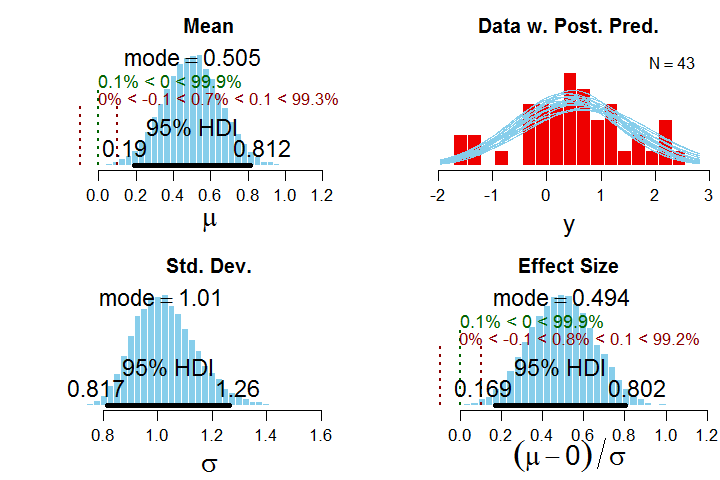Figure 1. Posterior when using unif(0,1000) prior on sigma, shown in Fig's 2 and 3.
First, consider the mu (mean) parameter. From the relation of the 95% HDI and ROPE, we would decide that a value of 0 for mu is not very credible, with the entire HDI outside the ROPE and only 0.7% of the posterior distribution practically equivalent to the null value. For the effect size, a similar conclusion is reached, with the 95% HDI completely outside the ROPE, and only 0.8% of the posterior practically equivalent to the null value. Note that the ROPEs for mu and effect size have been chosen here to be commensurate.

To determine Bayes factors (BFs) for mu and effect size, we need to consider the prior distribution in more detail. It has a broad normal prior on mu with an SD of 100 and a broad uniform prior on sigma from near 0 to 1000, as shown in Figures 2 and 3: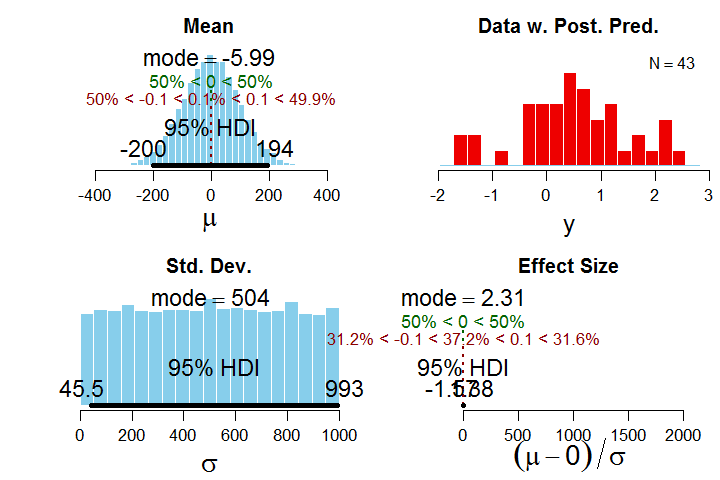Figure 2. Prior with unif(0,1000) on sigma.  Effect size is shown better in Figure 3.

The implied prior on the effect size, in the lower right above, is plotted badly because of a few outliers in the MCMC chain, so I replot it below in more detail: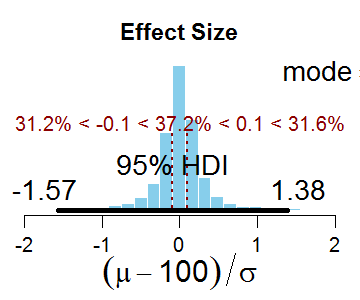Figure 3. Implied prior on effect size for unif(0,1000) prior on sigma.

The BF for a test of the null hypothesis on mu is the probability mass inside the ROPE for the posterior relative to the prior. In this case, the BF is 0.7% / 0.1% (rounded in the displays) which equals about 7. That is, the null hypothesis is 7 times more probable in the posterior than in the prior (or, more carefully stated, the data are 7 times more probable under the null hypothesis than under the alternative hypothesis). Thus, the BF for mu decides in favor of the null hypothesis.

The BF for a test of the null hypothesis on the effect size is the analogous ratio of probabilities in the ROPE for the effect size. The BF is 0.8% / 37.2% which indicates a strong preference against the null hypothesis. Thus, the BF for mu disagrees with the BF for the effect size.

Now we use a different vague prior on sigma, namely unif(0,10), but keeping the same vague prior on mu: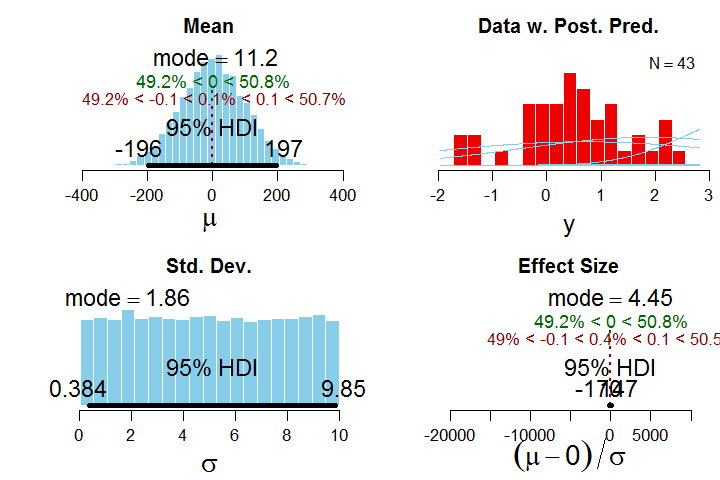Figure 4. Prior with unif(0,10) on sigma. Effect size is replotted in Figure 5. Compare with Figure 2.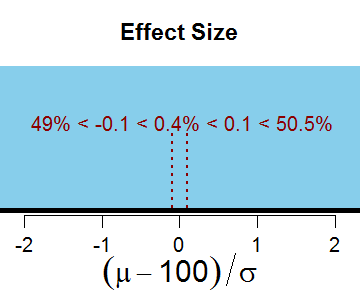Figure 5. Effect size replotted from Figure 4, using unif(0,10) on sigma.

The resulting posterior distribution looks like this: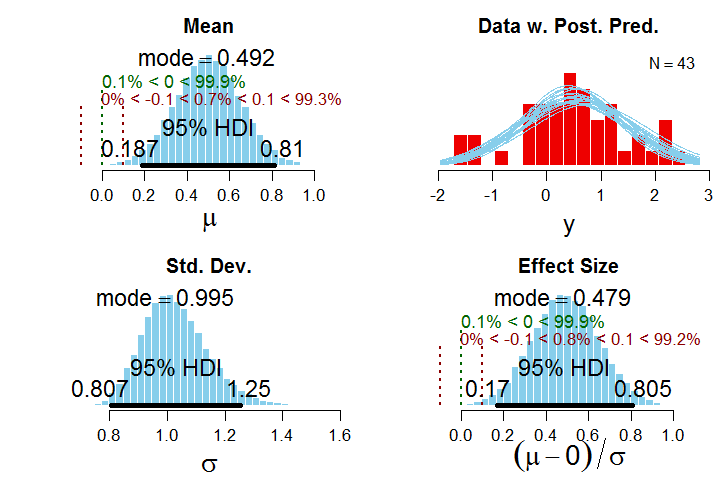Figure 6. Posterior when using unif(0,10) prior on sigma.
Compare the posterior in Figure 6 with the posterior in Figure 1. You will see they are basically identical. In other words, the 95% HDIs have barely changed at all, and decisions based on HDI and ROPE are identical, and the probability statements are identical.

But the BF for effect size is rather different than before. Now it is 0.8% / 0.4%, which is to say that the probability of the null hypothesis has gone up, i.e., this is a BF that leans in favor of the null hypothesis. Thus, a less vague prior on sigma has affected the implied prior on the effect size, which, of course, strongly affected the BF on effect size.

To summarize so far, a change in the breadth of the prior on sigma had essentially no effect on the HDIs of the posterior distribution, but had a big effect on the BF for the effect size while having no effect on the BF for mu.

Proponents of BFs will quickly point out that the priors used here are not well calibrated, i.e., they are too wide, too diluted. Instead, an appropriate use of BFs demands a well calibrated prior. (Proponents of BFs might even argue that an appropriate use of BFs would parameterize differently, focusing on effect size and sigma instead of mu and sigma.) I completely agree that the alternative prior must be meaningful and appropriate (again, see Ch. 12 of DBDA2E, or this article, or Appendix D of this article) and that the priors used here might not satisfy those requirements for a useful Bayes factor.

But there are still two take-away messages:
First, the BF for the mean (mu) need not lead to the same conclusion as the BF for the effect size unless the prior is set up just right.
Second, the posterior distribution on mu and effect size is barely affected at all by big changes in the vagueness of the prior, unlike the BF.